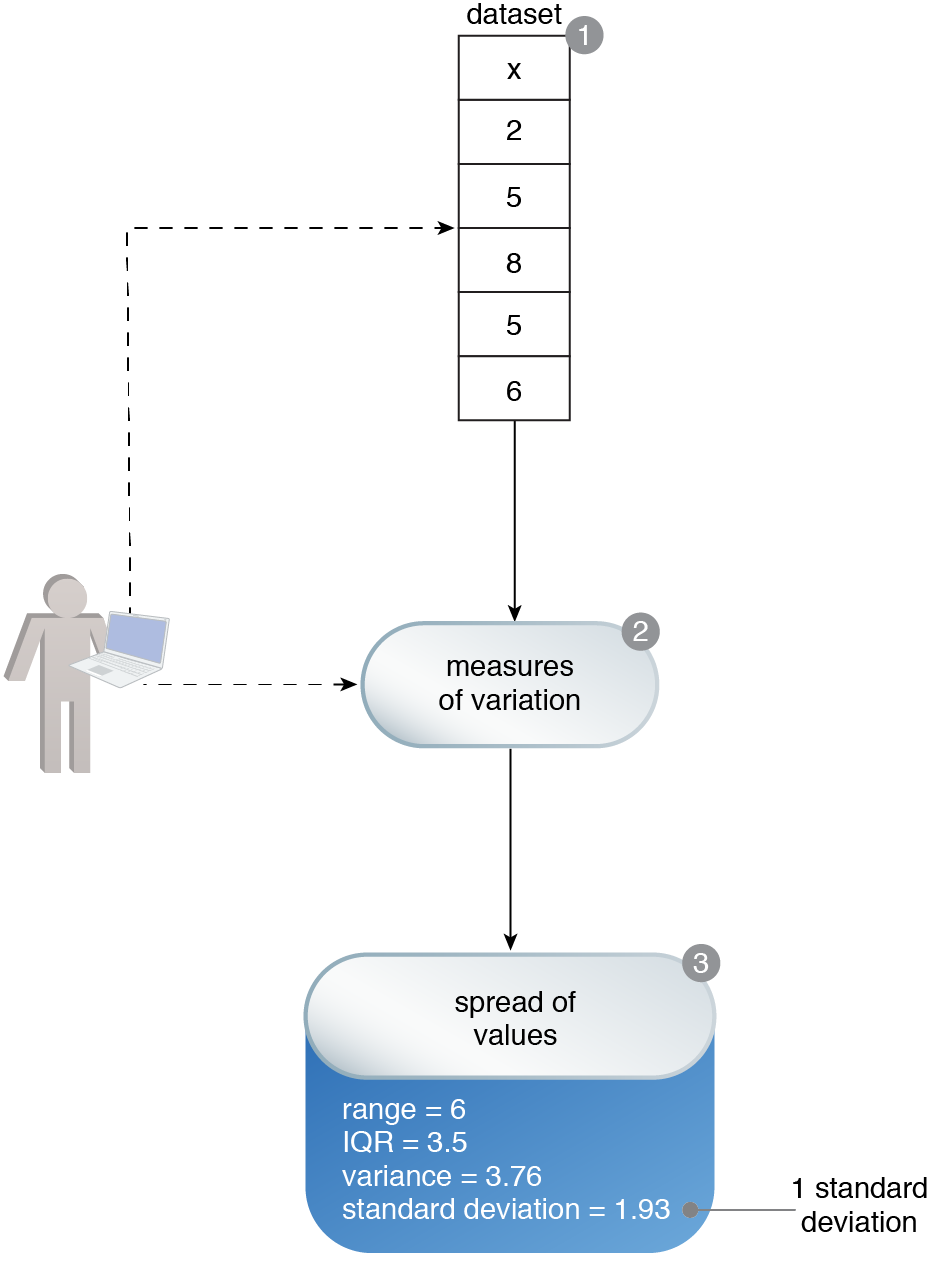# Variability Computation

Machine Learning Patterns, Mechanisms > Data Exploration Patterns > Variability Computation

## Variability Computation (Khattak)

How can the spread of values for a single variable in a dataset be determined?

### Problem

Developing an intuition about a dataset involves determining the behavior of uncommon values of data. Failure to do so may result in treating abnormal values as normal.

### Solution

The behavior of the uncommon values in a dataset is expressed in the form of the spread of values and is quantified via the application of proven statistical techniques.

### Application

The numerical values in the dataset are identified and measures of variation, including range, interquartile range (IQR), variance, and standard deviation, are calculated.

### Mechanisms

Query Engine, Analytics Engine, Processing Engine, Resource Manager, Storage DeviceVariable x belongs to a dataset. An understanding of the values of variable x is required. It is determined that the most common value is 5 (1). In order to gain insight into the behavior of the remaining values, the measures of variation are calculated (2). Based on the value of standard variation, it is determined that the average spread of values from the mean value is 1.93, and further notes that the value of 8 is beyond 1 standard deviation (1 standard deviation is 5.2 + 1.93 = 7.13, whereas 5.2 is the mean value).